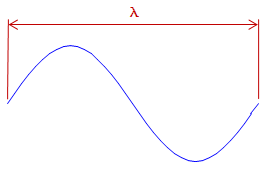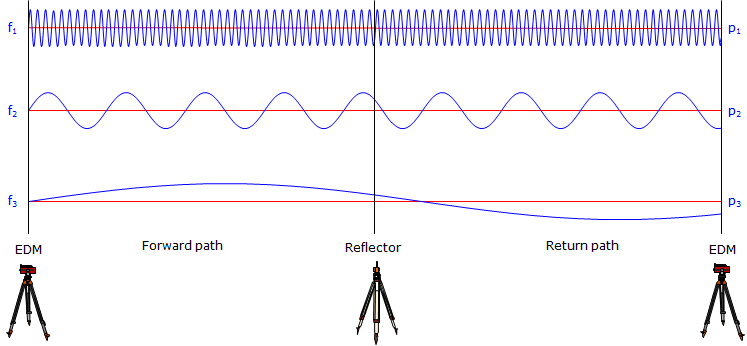## 1. Electromagnetic Energy

The electromagnetic principles of Electronic Distance Measurement (EDM) theory and operation are well covered in most Surveying text books and on the internet. The intent here is to give the reader a general understanding of EDM so that error sources are better understood and controlled.

An EDM uses electromagnetic (EM) energy to determine the length of a line. The energy originates at an instrument at one end of a line and is transmitted to a "reflector" at the other end from where it is returned to the originating instrument. The nature of the "reflector" is dependant on the type of EM.

If electro-optical (infrared or laser) EM is used, Figure A-1, then the "reflector" is typically a passive device which bounces the signal back to the transmitter.

If the EM is microwave, Figure A-2, then the reflector is a second instrument which captures the incoming energy and re-transits it back to the originating instrument.Figure A-1 Electro-optical EDMFigure A-2 Microwave EDM

In either case the measurement is the total distance from the instrument to the reflector and back to the instrument.

We will limit discussion to electro-optical EM instruments since the majority of EDMs and Total Stations employ that EM type.

## 2. Distance Determination

An EDM uses the EM signal structure and determines distance using a phase shift. The EM signal has a sinusoidal wave form. Remember from trigonometry that the sine curve looks like, Figure A-3:Figure A-3 Sine Curve

This wave form repeats every 360°. The distance between wave form ends is the wavelength, λ, Figure A-4.Figure A-4 Wavelength

Different wavelengths are generated at different modulation frequencies, f. Wavelength, frequency, and the speed of light are related by:

 λ = c/f Equation A-1 λ: wavelength c: speed of light f: frequency

The wavelength is a known quantity since it is generated by the EDM at a specific frequency. The signal leaves the EDM at 0° phase, goes thru N number of full phases on its path to then from the reflector, and returns to the EDM at some angle between 0° and 360° creating a partial wavelength, p, Figure A-5:Figure A-5 Phase Shift

The EDM can very accurately determine the length of the last partial wavelength from its phase. The total EDM-reflector-EDM distance is (Nλ + p).

Example
The wavelength in Figure A-5 is 20.00 ft.There are 10 full wavelengths before the last partial one. What is the EDM to reflector distance?

The last partial wave is: p = (82°30'39.9"/360°00'00") x 20.00 ft = 4.584 ft

Including the full wavelengths the total distance EDM-reflector-EDM is: 10 x 20.00 ft + 4.854 ft = 204.584 ft

The distance between the EDM and reflector is half that: 204.584 ft / 2 = 102.292 ft.

Unfortunately, while the EDM can accurately measure the last partial wavelength, it doesn't know how many full wavelengths occurred before it (this is similar to the integer ambiguity in carrier-based GPS measurements).

So how does the EDM resolve this dilemma?

By decreasing the frequency by a factor of 10 and repeating the process. Decreasing the frequency by a factor of 10 increases the wavelength by a like amount. The partial wavelength at this level will give the next higher distance digit. This is repeated a number of times until the distance is resolved.

Figure A-6 illustrates three frequencies each folded out to show a continuous EDM-reflector-EDM path:Figure A-6 Multiple Frequencies

Example

The follwing table shows the last partial length for each of 4 different wavelengths. What is the total distance EDM-reflector?

 λ, m p, m dist, m 10.00 3.68 100.0 53.7 1,000 454 10,000 8450

The digits in bold represent the digits added to the distance as a result of each partial wavelength.

 λ, m p, m dist, m 10.00 3.68 3.68 100.0 53.7 53.68 1,000 454 453.68 10,000 8450 8453.68

The total distance is 8456.68 m; the EDM-reflector distance is 8453.68/2 = 4226.84 m

## 3. Distance Reduction

An EDM measures the line of sight distance between the instrument and reflector. This is a slope distance. Figure A-8, and not horizontal unless the EDM and reflector are at the same elevation.Figure A-7 Slope Measurement

In order to determine a horizontal or vertical distance additional information is needed. Combining an EDM with a digital theodolite results in a Total Station Instrument (TSI). The TSI measures the slope distance and a zenith angle from which it computes a horizontal and vertical distance, Figure A-8..Figure A-8 Slope Reduction

 H = S x sin(Z) Equation A-2 V - S x cos(Z) Equation A-3

It's a little more complex than this and we'll discuss a refinement in the Errors chapter.

## 4. Evolutionary sidebar

Early attempts to integrate EDMs with theodolites resulted in some pretty interesting (and bizarre) hybrid instruments.

The first affordable EDMs were stand alone and could only measure distances - no zenith angles. A typical procedure is shown in Figure A-9:

(1) measure a zenith angle with a theodolite,

(2) remove the theodolite from the tripod and mount the EDM (often using the same tribrach to maintain the same setup) and measure the slope distance, and, finally

(3) manually reduce the slope distance to horizontal.Figure A-9 Swapping Instruments

As EDMs became more affordable and smaller, other integration methods appeared.

EDMs were placed in yokes mounted to a theodolite's standards, Figure A-10(a). The vertical angle would be measured with the theodolite, and recorded or manually entered into the EDM. The slope distance would be measured with the EDM. Slope would be reduced to horizontal either manually or by the EDM if it could accept angle input.(a) Standards Mount (b) Convergence Figure A-10 Standards Mount EDM

The advantage of this mounting method was that the EDM's measuring center was always vertically above the same point - it did not change position as it was elevated or depressed to sight the prism.

The disadvantage was that if the zenith angle was measured to the center of the prism an offset error was introduced because the signal path and line of sight weren't coincident, Figure A-10(b)

Another mounting method placed the EDM on top of, Figure A-11, and later wrapped around the theodolite telescope. Measurement and slope reduction was similar to that of a yoke-mount EDM.This method had the same disadvantage as the yoke mount plus two additional ones:

(1) It shifted the measuring center of the EDM as the zenith angle changed (necessitating more computations), and,

(2) it stressed the telescope mount and lock which were not designed for the additional eccentric weight.Figure A-11 Telescope Mount EDM

It wasn't until the digital theodolite was developed that the EDM could be seamlessly integrated with an angle measuring device: the Total Station. This has become the primary instrument for most surveyors and represents the latest evolutionary step of the EDM. For the rest of this chapter, we will discuss distance measurement with a TSI.

## 5. Reflector

Any surface capable of reflecting the electro-optical signal will allow distance measurement. However, the more efficient the reflector, the stronger the returned signal and the longer distance which can be measured. Efficiency includes amount of signal reflected along with the direction of its return path. For example, while a flat mirror reflects most of the signal, Figure A-12(a), if it is not perpendicular to the incoming path the signal will be reflected away from the TSI, Figure A-12(b).(a) Perpendicular to Signal (b) Angled to Signal Figure A-12 Using a Mirror

To overcome this problem, a corner cube prism is used as a reflector for most TSIs. A corner cube prism is based on a 45° right angle prism. This type of prism has the property that any signal which intersects its long (hypotenuse) side will be reflected parallel to the incoming path even if the prism is not perpendicular to the signal path, Figures A-13(a) and (b).(a) Perpendicular to Signal (b) Angled to Signal Figure A-13 Right Angle Prism

A typical corner cube prism uses a glass cylinder having three 45° facets at one end. This creates three right angle prisms all sharing the glass cylinder's flat front as their hypotenuse. From the front the facets appear as six radial segments, Figure A-14.Figure A-14 Corner Cube Prism

The result is a highly efficient reflector for both signal strength and direction. Efficiency can be increased by using multiple prisms, Figure A-15. This results in more signal being reflected increasing distance range. Using a triple prism can increase range by 50-60% depending on atmospheric conditions.Figure A-15 Prism Array

Over short distances of a few hundred feet, other objects such as bicycle reflectors and reflective tape will also work. While not as efficient as a prism they have the advantage of being inexpensive.

We will use the terms reflector and prism interchangeably in subsequent discussions.

## 6. Reflectorless Total Stations

The past decade has seen the introduction and maturation of reflectorless total stations. Their inherent advantage is the ability to measure distances to points not accessible with a prism. Their biggest drawback is their generally (much) shorter range. This is in large part dependent on surface reflectivity. However, most reflectorless instruments can also use a prism as a conventional TSI giving them greater flexibility.

A reflectorless TSI uses short pulses of high energy laser light. This energy is considerably higher than that used by phase shift TSIs in order to get a return signal off low reflection surfaces. The instrument measures travel times of the laser pulses and from that can determine the total instrument-surface-instrument distance.

Because the laser pulses reflect off different surfaces, care must be exercised when pointing the instrument. This is especially critical when there are multiple surfaces at various orientations near the measurement point. Many instruments feature a built-in laser pointer which provides the operator a visual indication of where the measurement will be made.

Hits: 53589